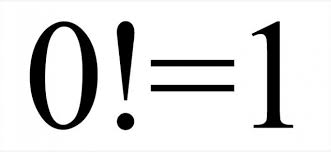Saturday, 30 May 2015

How to play War+ Card Game

How to play the "War+" Card Game:

Age: 5+
Players: 2 only
Deck size: 54 or 52 cards
Setup: Deal all cards between the two players

Goal: Be the first player to have all 52 cards

How to play:
One player starts by putting down the top card of his/her deck. You are not allowed to look at the card until it's down. Then the other player puts down a card and the one with the highest score wins. Each round the score resets.
Note: Each time a card is put down the card score is added to the players score.

Thursday, 21 May 2015

Why n⁰ = 1

This is why I think anything⁰ = 1
Just another way that seems to work and make sense.

x=4
x⁴ = 256
x³ = 64
x³ is also = x⁴/x
x² = 16
x² is also = x³/x
x¹ = 4
x¹ is also = x²/x

So according to what worked above ^^, I will assume it will work for 0 too:
x⁰ = x¹/x = 4/4 = 1

Try this out with any number, and it should work.
If the number is below 0 it will give -1.

I found this out myself and posted it to http://david.callanan.ie
Afterwards I got proof from here: http://mathforum.org/library/drmath/view/58233.html

Wednesday, 20 May 2015

Why 0! = 1

Why 0 Factorial (0!) is equal to 15! = 5*4*3*2*1 = 120
4! = 4*3*2*1 = 24

4! is also = 5! / 5, because 4! has all in 5! but the five, so simply divide by five. Now let's continue a bit more..

3! = 4!/4 = 6
3! = 3*2*1 = 6
2! = 3!/3 = 2
2! = 2*1 = 2
1! = 2!/2 = 1
1! = 1 = 1

Now if you look at 0!, this is the reason it is equal to 1..

0! = 1!/1 which is 1/1, which is 1
therefore 0! is 1

You can also go as far as -1! if you'd like
-1! = 0!/0 which is 1/0
-1! = undefined, infinity, etc..

I found this out myself and posted to http://david.callanan.ie/
I then found proof here: http://mathforum.org/dr.math/faq/faq.0factorial.html

Saturday, 16 May 2015

How 0.9999... = 1

Here's two proofs of why 0.9999999... is equal to 1

Proof 1:

p = 0.9999...
10p = 9.9999...
9p = 10p - p
9p = 9
p = 1

p started as 0.9999... but is now 1.

Proof 2:

1/3 = 0.333333...
2/3 = 0.666666...

1/3 + 2/3 = 1
0.333333... + 0.666666... = 0.999999...

Therefore 0.9999... = 1